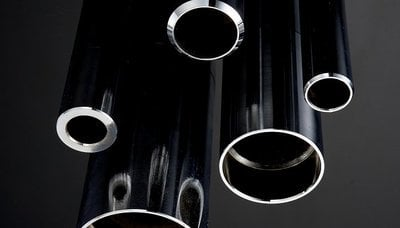# Section Modulus Calculator Update: Tube Bending Calculations

May 23, 2016 by
Mike Thomas

Earlier in the spring we offered our readers a Section Modulus Calculator to help with calculating the section area moment of inertia properties of common shapes for tube bending and fabricating metal. This calculator was so popular among our readers that we've decided to update the calculator to include more formulas used for tube bending.The original calculator allowed you to input the tube specifications and calculated the section area moment of inertia properties of common shapes. The shapes covered by the original calculator included:

• Square
• Round
• Half rounds
• Triangles
• Rectangles
• Trapezoids
• Hexagons
• Octagons

The updated Section Modulus Calculator will now also include a range of formulas for tube bending in the same easy to use format! The updated calculator will offer a list of all formulas used for tube bending!